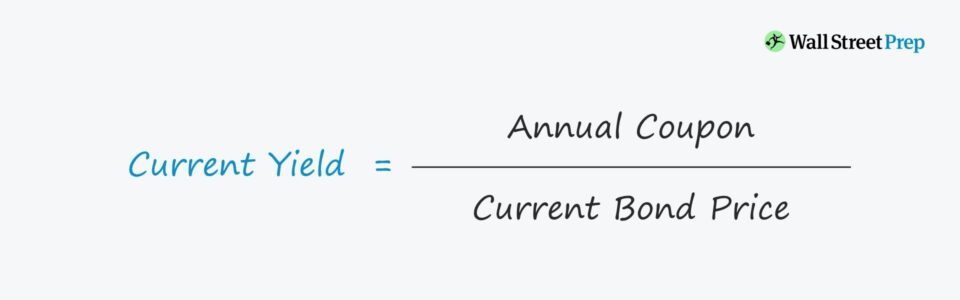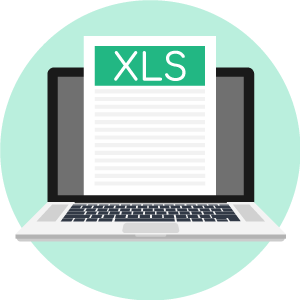# Current Yield

Guide to Understanding the Current Yield Concept• What is the current yield formula?
• What are the limitations to the current yield?
• How is the current yield different from yield-to-maturity (YTM)?
• What are the steps to calculating the current yield?

## Current Yield Formula

The current yield on a bond depicts the annual coupon as a percentage of the market price – which could be higher or lower than par.

The current yield is the bondholder’s rate of return if the investment is held for the next year.

The calculation of current yield is a straightforward 3-step process:

• Step 1: First, the current market price of the bond can be readily observed – in which the bond can either trade at a discount, at par, or a premium to par.
• Step 2: Next, the annual coupon must be calculated, which is a function of the bond’s coupon rate, par value, and payment frequency, if applicable (i.e. the coupon rate must be annualized).
• Step 3: Finally, the current yield formula is equal to the annual coupon payment divided by the bond’s current market price, expressed as a percentage.

### Current Yield Formula

• Current Yield = Annual Coupon ÷ Bond Price

For instance, if a corporate bond with a \$1,000 face value (FV) and an \$80 annual coupon payment is trading at \$970, then its current yield equals 8.25%.

• Current Yield = \$80 Annual Coupon ÷ \$970 Bond Price
• Current Yield = 8.25%

## Current Yield of Discount, Par & Premium Bonds

The difference between the current yield and coupon rate of a bond stems from the pricing of the bond diverging from its par value.

In practice, the current yield is a simple, convenient metric, yet its utility tends to be limited in scope.

The current yield is most often relevant only if the market price deviates from its par value.

• Bond Price < Par Value → “Discount” Bond
• Bond Price = Par Value → “Par” Bond
• Bond Price > Par Value → “Premium” Bond

Since the price of a bond adjusts based on the prevailing macro conditions and news surrounding the underlying issuer, bonds can be purchased at discounts or premiums relative to par.

• If a bond is trading below its face value (FV), the current yield is higher than the coupon rate.
• If a bond is trading at its face value (FV) – i.e. “at par” – the current yield is equivalent to the coupon rate.
• If a bond is trading in excess of its face value, the current yield is lower than the coupon rate.

## Current Yield vs Yield-to-Maturity (YTM)

The yield-to-maturity (YTM) is the annualized return expected to be earned on a bond, assuming that the bond is held until the date of maturity.

Moreover, YTM is the internal rate of return (IRR) on the bond and is widely considered a far more useful measure for comparisons among different bonds.

One criticism of YTM is that all coupons are implicitly presumed to be received on time and reinvested at the same interest rate.

The current yield entirely disregards the effects of principal repayment and reinvestment – therefore, the current yield is not a sufficient standalone measure of the actual yield on a bond.

• The current yield inflates the return of premium bonds, i.e.  bonds trading at above their par value.
• By contrast, the return implied by the current yield is understated for discount bonds, i.e. trading at below their par value.

Why? The only income source considered under the calculation of the current yield is the anticipated annual coupon payment – while neglecting reinvestment risks, capital gains/losses, compounding, and the time value of money.

## Current Yield Calculator – Excel Template

We’ll now move to a modeling exercise, which you can access by filling out the form below.Submitting ...

## Bond Current Yield Example Calculation

In our illustrative scenario, we’ll assume three bonds were each issued at a face (par) value of \$1,000 with an annual coupon rate of 6%.

• Face Value of Bond (FV) = \$1,000
• Coupon Rate (%) = 6.00%

Since the annual coupon depends on the bond’s original face value (FV), the coupon can be calculated by multiplying the coupon rate by the FV of the bond.

• Annual Coupon = 6.00% × \$1,000
• Annual Coupon = \$60

However, the current market prices of the bonds are all different, with the bonds trading below par, at par, and above par, respectively:

• Discount Bond = \$950
• Par Bond = \$1,000

For each bond, the current yield is equal to the annual coupon divided by the bond’s face value (FV).

• Discount Bond = \$60 ÷ \$950 = 6.32%
• Par Bond = \$60 ÷ \$1,000 = 6.00%
• Premium Bond = \$60 ÷ \$1,050 = 5.71%

If a bond is trading at par, the current yield is equal to the stated coupon rate – thus, the current yield on the par bond is 6%.

But for the discount bond, the current yield (6.32%) is higher than the coupon rate, whereas the opposite is true for the premium bond (5.71%).

Conversely, another method to calculate the current yield is to divide the coupon rate by the bond quote (% of par) – with the result then multiplied by 100.Globally Recognized Certification Program

#### Get the Equities Markets Certification (EMC©)

This self-paced certification program prepares trainees with the skills they need to succeed as an Equities Markets Trader on either the Buy Side or Sell Side.